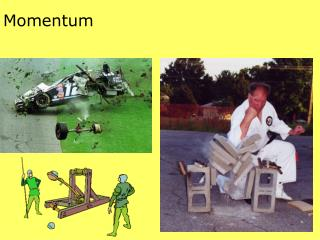DownloadDownload PresentationMomentum

# Momentum

Download Presentation## Momentum

- - - - - - - - - - - - - - - - - - - - - - - - - - - E N D - - - - - - - - - - - - - - - - - - - - - - - - - - -
##### Presentation Transcript

1. Momentum

2. Momentum—Inertia in Motion 22 km/h 5225 kg 75 km/h 1540 kg • Momentum = mass x velocity or momentum = mv Which has greater momentum?

3. Impulse Changes Momentum Egg drop challenge The greater the force acting on an object, the greater its velocity and momentum. Time is also a factor in determining momentum. How long is force applied? Impulse = force x time interval orimpulse = Ft Impulse = change in momentum Note: The difference between impulse and impact force involves the time the force acts.

4. Increasing Momentum To increase momentum, force must be applied as hard as possible for as long as possible. This is why follow through is important in a golf swing or in baseball. Large force applied for a long time= maximum impulse Impact measured in N. Impulse measured in N-s

5. Decreasing Momentum To decrease the force of impact, the impact time (time during which momentum is brought to zero) must be lengthened.

6. Decreasing Momentum To decrease the force of impact, the impact time (time during which momentum is brought to zero) must be lengthened.

7. A 0.50-kg cart (#1) is pulled with a 1.0-N force for 1 second; another 0.50 kg cart (#2) is pulled with a 2.0 N-force for 0.50 seconds. • Which cart (#1 or #2) has the greatest acceleration? Explain. • Which cart (#1 or #2) has the greatest impulse? Explain. • Which cart (#1 or #2) has the greatest change in momentum? Explain.

8. Decreasing Momentum

9. Decreasing Momentum

10. Decreasing Momentum

11. Decreasing Momentum Momentum (mv) is what is gained by the Jumper until the chord begins to stretch.

12. Decreasing Momentum Momentum (mv) is what is gained by the Jumper until the chord begins to stretch. Ft is the impulse the cord supplies to reduce the momentum to zero. Because of the long time it takes the cord to stretch, the average force (F) on the jumper is minimal.

13. Decreasing Momentum HELP!!!

14. Bouncing Impulses are greater when an object bounces. Flower pot on the head Pelton Wheel

15. Conservation of Momentum • Law of conservation of momentum: In the absence of an external force, the momentum of a system remains unchanged. The bullet gains momentum and so does the rifle, but the rifle-bullet system gains none.

16. Collisions • When objects collide in the absence of external forces, the net momentum of both objects before the collision equals the net momentum of both objects after the collision. net momentum before collision = net momentum after collision Elasticcollision—when objects collide without being deformed permanently or generating heat. Inelasticcollision—when colliding objects become tangled or coupled together

17. Elastic Collisions • The sum of the momentum vectors are the same before and after the collisions.

18. Inelastic Collisions V = 12 m/s V = 0 • If mass is equal, the momentum is shared equally after the collision by each of the objects. V = 6 m/s

19. Inelastic Lunch 3 m/s 20 kg 5 kg v = 0

20. Inelastic Lunch 5 kg 25 kg 2.4 m/s 3 m/s 20 kg (net mv) before = (net mv) after (20 kg)(3 m/s)+(5 kg)(0 m/s) = (25 kg)(v after) (60 kg m/s)+(0) = (25 kg)(v after) (60 kg m/s) = (25 kg)(v after) 2.4 m/s = (v after)

21. Inelastic Lunch 5 kg 3 m/s 20 kg 2 m/s (net mv) before = (net mv) after (20 kg)(3 m/s)+(5 kg)(-2 m/s) = (25 kg)(v after) (60 kg m/s)+(-10 kg m/s) = (25 kg)(v after) (50 kg m/s) = (25 kg)(v after) 2 m/s = (v after)

22. Inelastic Lunch 5 kg v = 0 (net mv) before = (net mv) after (20 kg)(3 m/s)+(5 kg)(-2 m/s) = (25 kg)(v after) (60 kg m/s)+(-10 kg m/s) = (25 kg)(v after) (50 kg m/s) = (25 kg)(v after) 2 m/s = (v after) 2 m/s 25 kg

23. Inelastic Lunch 5 kg 10 m/s 3 m/s 20 kg (net mv) before = (net mv) after (20 kg)(3 m/s)+(5 kg)(-10 m/s) = (25 kg)(v after) (60 kg m/s)+(-50 kg m/s) = (25 kg)(v after) (10 kg m/s) = (25 kg)(v after) .4 m/s = (v after)

24. Inelastic Lunch 5 kg v = 0 (net mv) before = (net mv) after (20 kg)(3 m/s)+(5 kg)(-10 m/s) = (25 kg)(v after) (60 kg m/s)+(-50 kg m/s) = (25 kg)(v after) (10 kg m/s) = (25 kg)(v after) .4 m/s = (v after) .4 m/s 25 kg

25. Inelastic Lunch Solve this problem on a sheet of loose leaf paper. Show all work. Write the answer at the bottom along with the appropriate velocity vector. 50 kg 10 kg 7 m/s 5 m/s

26. Momentum Vectors (diagonal of a square)